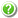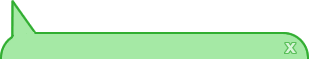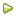[Home|New job|Help]

The RNAprobing web server will predict a vector of perturbation energies which minimizes the differences between predicted and observed pairing probabilities. Current limit is 1000nt.

Simply paste or upload your sequence below and click Proceed. To get more information on the meaning of the options click thesymbols. You can test the server using this sample data.

 Paste or type your sequence here: [clear]

 Paste or type your SHAPE reactivities here: [clear]

Fold algorithms and basic options
 Deigan et al. 2009 Zarringhalam et al. 2012 Washietl et al. 2012 SHAPE methodDetermines which method is used to incorporate guiding pseudo energies into the folding algorithm. Pseudo energies can be applied to stacked pairs (Mathews et al.) or to all positions disagreeing with pairing probabilities derived from probing data (Zarringhalam et al.). The dynamic generation of a vector of perturbation energies yields additional information about nucleotides where prediction and probing data disagree (Washietl et al.). Linear Mapping Cutoff Identity (Skip normalization) Linear model Linear log model Method used to derive pairing probabilitiesSince the choosen method operates requires experimentally determined pairing probabilities as input data, the probing data for each nucleotide has to be converted into a certain probability for being unpaired using one of the folling methods: Linear Mapping: Map various reactivity ranges to probability ranges according to Zarringhalam et al. Cutoff: Use a cutoff-approach to divide into paired and unpaired nucleotides. Identity (Skip Normalization): Skip the normalizing step since the input data already represents probabilities for being unpaired rather than raw reactivity values. Linear model: Use a linear model to convert the reactivity into a probability for being unpaired. Linear log model: Use a linear model to convert the log of the reactivity into a probability for being unpaired. cutoff threshold: slope (s) intercept (i) slope (s) intercept (i) Additional parameters for the method defined aboveAdditional parameters for the selected conversion: Cutoff: Cutoff value used to divide into paired and unpaired nucleotides. Linear model: Slope and intercept used to perform the linear conversion. beta (b)The factor beta directly influences the magnitude of the penalty for mismatches with experimental data. Setting beta to 0 will result in the same prediction as obtained when using no soft constraints at all. With growing values of beta, discrepancies with the experimental data become more and more costly and the results tend to converge to the experimentally determined pattern. slope (m)Slope/Intercept used for the linear conversion from SHAPE reactivities to pseudo energies. intercept (b)Slope/Intercept used for the linear conversion from SHAPE reactivities to pseudo energies. ratio between the weighting factors tau and sigmaThe weighting factors sigma and tau determine the impact of energy model adjustments and discrepancies between predicted and observerd pairing probabilities onto the objective function score. Choosing a high tau/sigma-ratio will lead to results close to the experimentally determined pairing pattern while a low tau/sigma-ratio will lead to results close to the thermodynamic prediction without guiding pseudo energies. Gradient descent GSL Fletcher-Reeves conjugate gradient GSL Polak-Ribiere conjugate gradient GSL vector Broyden-Fletcher-Goldfarb-Shanno GSL vector Broyden-Fletcher-Goldfarb-Shanno 2 GSL steepest descent minimizer algorithmThe algorithm used to minimize the objective function. Choose between a custom implementation of the gradient descent algorithm and several multidimensional minimizer algorithms implemented in the GNU Scientific Library. limit for vector initialisationValue range for random initialization of the initial vector (i.e. a value of 0.1 will initialize the perturbation vector with random values between -0.1 and 0.1 kcal/mol. Use the value 0 to start the minimization process with a null vector. number of samples used to estimate pairing probabilitiesThe amount of sampled structures used to estimate pairing probabilities. A value <= 0 will force the exact evaluation of the pairing probabilities.Show advanced options

Notification via e-mail upon completion of the job (optional):

Underlying principlies can be found in:
Katherine E. Deigan, Tian W. Li, David H. Mathews, Kevin M. Weeks Accurate SHAPE-directed RNA structure determination. PNAS, 106:97-102, 2009.
Kourosh Zarringhalam, Michelle M. Meyer, Ivan Dotu, Jeffrey H. Chuang, Peter Clote. Integrating Chemical Footprinting Data into RNA Secondary Structure Prediction. PLOS ONE, 7(10), 2012.
Stefan Washietl, Ivo L. Hofacker, Peter F. Stadler, Manolis Kellis. RNA folding with soft constraints: reconciliation of probing data and thermodynamics secondary structure prediction. Nucleic Acids Research, 40(10):4261-4272, 2012.